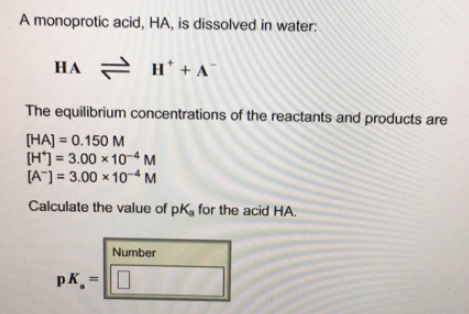# Problem: A monoprotic acid, HA, is dissolved in water HA ⇌ H+ + A- The equilibrium concentrations of the reactants and products are HA 0.150 M [H+] = 3.00 × 10-4 M[A-] = 3.00 × 10-4 M Calculate the value of pka for the acid HA.

###### FREE Expert Solution

Ka or acid dissociation constant can be expressed using the reaction equation as:

80% (491 ratings)###### Problem Details

A monoprotic acid, HA, is dissolved in water

HA ⇌ H+ + A-

The equilibrium concentrations of the reactants and products are

HA 0.150 M

[H+] = 3.00 × 10-4 M

[A-] = 3.00 × 10-4

Calculate the value of pka for the acid HA.